MNE : Demo of multivariate statistics (decoding / MVPA)¶

Author : Alexandre Gramfort

In :
# add plot inline in the page
%matplotlib inline


In :
import mne


We set the log-level to 'WARNING' so the output is less verbose

In :
mne.set_log_level('WARNING')


Access raw data¶

Now we import the sample dataset. If you don't already have it, it will be downloaded automatically (but be patient approx. 2GB)

In :
data_path = '/Users/alex/Sync/karolinska_teaching/'
raw_fname = data_path + '/meg/workshop_visual_sss.fif'

print raw_fname

/Users/alex/Sync/karolinska_teaching//meg/workshop_visual_sss.fif



In :
raw = mne.fiff.Raw(raw_fname)
print raw

<Raw  |  n_channels x n_times : 322 x 988000>



!!! we need to fix now tiny acquisition problems

In :
def fix_info(raw):
raw.info['chs'][raw.ch_names.index('BIO001')]['kind'] = mne.fiff.constants.FIFF.FIFFV_EOG_CH
raw.info['chs'][raw.ch_names.index('BIO002')]['kind'] = mne.fiff.constants.FIFF.FIFFV_EOG_CH
raw.info['chs'][raw.ch_names.index('BIO003')]['kind'] = mne.fiff.constants.FIFF.FIFFV_ECG_CH

fix_info(raw)

In :
print raw.info['bads']

[]



First extract events:

In :
events = mne.find_events(raw, stim_channel='STI101', verbose=True)

Reading 0 ... 987999  =      0.000 ...   987.999 secs...
[done]
1473 events found
Events id: [10 11 12 13 14 16 17 20 21 22 23 24 26 27]



In :
plt.plot((events[:, 0] - raw.first_samp) / raw.info['sfreq'], events[:, 2], '.');
plt.xlabel('time (s)')
plt.axis([0.1168, 41.4003, 9.7295, 17.3887])

Out:
[0.1168, 41.4003, 9.7295, 17.3887]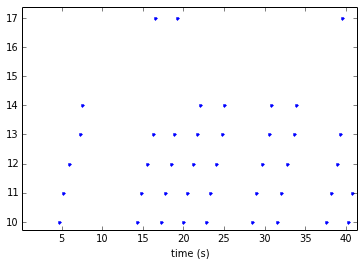From raw to epochs

Define epochs parameters:

In :
event_id = dict(left=14, right=24)  # event trigger and conditions
tmin = -0.3  # start of each epoch
tmax = 0.5  # end of each epoch
baseline = (-0.3, -0.15)

picks = mne.fiff.pick_types(raw.info, meg='grad', eeg=False, eog=True,

epochs = mne.Epochs(raw, events, event_id, tmin, tmax, proj=True,
picks=picks, baseline=baseline, reject=reject,
print epochs

<Epochs  |  n_events : 111 (all good), tmin : -0.3 (s), tmax : 0.5 (s), baseline : (-0.3, -0.15),
'left': 62, 'right': 49>


In :
epochs.plot_drop_log()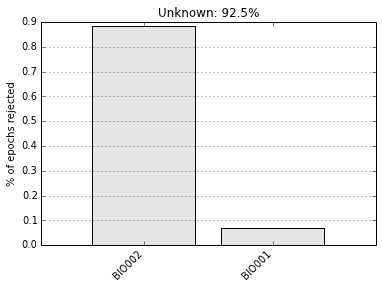Out:
92.464358452138498


Look at the ERF and contrast between left and rigth response

In :
evoked_left = epochs['left'].average()
evoked_right = epochs['right'].average()
evoked_contrast = evoked_left - evoked_right

In :
fig = evoked_left.plot()
fig = evoked_right.plot()
fig = evoked_contrast.plot()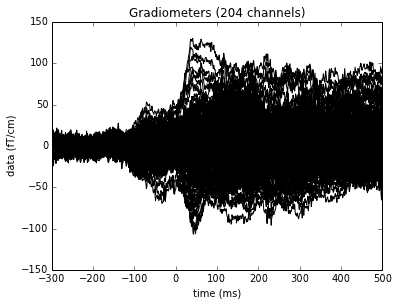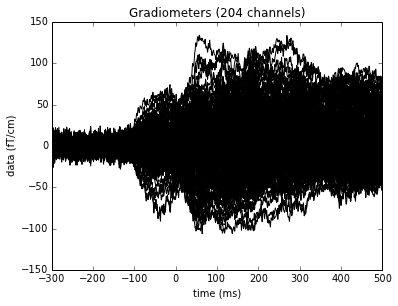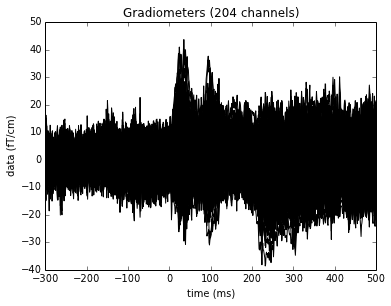Plot some topographies

In :
import numpy as np
times = np.linspace(-0.1, 0.5, 10)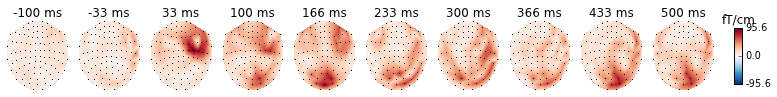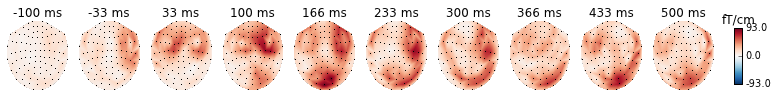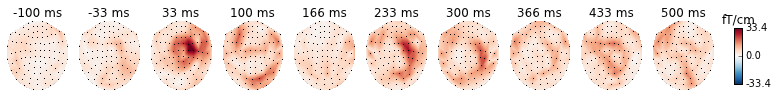Now let's see if we can classify single trials with an SVM

To have a chance at 50% accuracy equalize epoch count in each condition

In :
epochs_list = [epochs[k] for k in event_id]
mne.epochs.equalize_epoch_counts(epochs_list)


A classifier takes as input an x and return y (-1 or 1). Here x will be the data at one time point on all gradiometers (hence the term multivariate). We work with all sensors jointly and try to find a discriminative pattern between 2 conditions to predict the class.

In :
n_times = len(epochs.times)

# Take only the data channels (here the gradiometers)

# Make arrays X and y such that :
# X is 3d with X.shape is the total number of epochs to classify
# y is filled with integers coding for the class to predict
# We must have X.shape equal to y.shape

X = [e.get_data()[:, data_picks, :] for e in epochs_list]
y = [k * np.ones(len(this_X)) for k, this_X in enumerate(X)]
X = np.concatenate(X)
y = np.concatenate(y)

In :
print X.shape, y.shape
print y

(98, 204, 801) (98,)
[ 0.  0.  0.  0.  0.  0.  0.  0.  0.  0.  0.  0.  0.  0.  0.  0.  0.  0.
0.  0.  0.  0.  0.  0.  0.  0.  0.  0.  0.  0.  0.  0.  0.  0.  0.  0.
0.  0.  0.  0.  0.  0.  0.  0.  0.  0.  0.  0.  0.  1.  1.  1.  1.  1.
1.  1.  1.  1.  1.  1.  1.  1.  1.  1.  1.  1.  1.  1.  1.  1.  1.  1.
1.  1.  1.  1.  1.  1.  1.  1.  1.  1.  1.  1.  1.  1.  1.  1.  1.  1.
1.  1.  1.  1.  1.  1.  1.  1.]



For classification we will use the scikit-learn package (http://scikit-learn.org/)

In :
from sklearn.svm import SVC
from sklearn.cross_validation import cross_val_score, ShuffleSplit

# Define an SVM classifier (SVC) with a linear kernel
clf = SVC(C=1, kernel='linear')


Define a monte-carlo cross-validation generator (to reduce variance):

In :
cv = ShuffleSplit(len(X), 10, test_size=0.2, random_state=42)


The goal is going to be to learn on 80% of the epochs and evaluate on the remaining 20% of trials if we can predict accuratly.

In :
X_2d = X.reshape(len(X), -1)
X_2d = X_2d / np.std(X_2d)
scores_full = cross_val_score(clf, X_2d, y, cv=cv, n_jobs=1)
print "Classification score: %s (std. %s)" % (np.mean(scores_full), np.std(scores_full))

Classification score: 0.92 (std. 0.0458257569496)



It's also possible to run the same decoder and each time point to know when in time the conditions can be better classified:

In :
scores = np.empty(n_times)
std_scores = np.empty(n_times)

for t in range(n_times):
Xt = X[:, :, t]
# Standardize features
Xt -= Xt.mean(axis=0)
Xt /= Xt.std(axis=0)
# Run cross-validation
scores_t = cross_val_score(clf, Xt, y, cv=cv, n_jobs=1)
scores[t] = scores_t.mean()
std_scores[t] = scores_t.std()


A bit of rescaling

In :
times = 1e3 * epochs.times # to have times in ms
scores *= 100  # make it percentage accuracy
std_scores *= 100


Now a bit of plotting

In :
plt.plot(times, scores, label="Classif. score")
plt.axhline(50, color='k', linestyle='--', label="Chance level")
plt.axvline(0, color='r', label='stim onset')
plt.axhline(100 * np.mean(scores_full), color='g', label='Accuracy full epoch')
plt.legend()
hyp_limits = (scores - std_scores, scores + std_scores)
plt.fill_between(times, hyp_limits, y2=hyp_limits, color='b', alpha=0.5)
plt.xlabel('Times (ms)')
plt.ylabel('CV classification score (% correct)')
plt.ylim([30, 100])
plt.title('Sensor space decoding')

Out:
<matplotlib.text.Text at 0x1114c3810>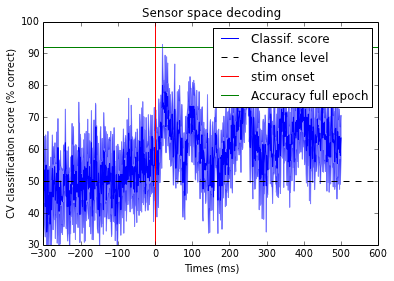Look at generalization over time

We can test how much the "decodability" stays over time.

Have a look at : http://martinos.org/mne/dev/auto_examples/decoding/plot_decoding_time_generalization.html

to get an idea of what to expect.

In :
from mne.decoding import time_generalization

# Compute Area Under the Curver (AUC) Receiver Operator Curve (ROC) score
# of time generalization. A perfect decoding would lead to AUCs of 1.
# Chance level is at 0.5.
# The default classifier is a linear SVM (C=1) after feature scaling.
scores = time_generalization(epochs_list, clf=None, cv=5, scoring="roc_auc",
shuffle=True, n_jobs=4)

/Users/alex/local/lib/python/site-packages/sklearn/cross_validation.py:1212: DeprecationWarning: check_cv will return indices instead of boolean masks from 0.17
/Users/alex/local/lib/python/site-packages/sklearn/cross_validation.py:62: DeprecationWarning: The indices parameter is deprecated and will be removed (assumed True) in 0.17
stacklevel=1)



Now visualize

In :
times = 1e3 * epochs.times  # convert times to ms

plt.imshow(scores, interpolation='nearest', origin='lower',
extent=[times, times[-1], times, times[-1]],
vmin=0., vmax=1.)
plt.xlabel('Times Test (ms)')
plt.ylabel('Times Train (ms)')
plt.title('Time generalization (%s vs. %s)' % tuple(event_id.keys()))
plt.axvline(0, color='k')
plt.axhline(0, color='k')
plt.colorbar()

Out:
<matplotlib.colorbar.Colorbar instance at 0x113b335a8>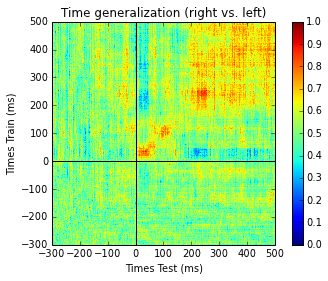In :
plt.plot(times, np.diag(scores), label="Classif. score")
plt.axhline(0.5, color='k', linestyle='--', label="Chance level")
plt.axvline(0, color='r', label='stim onset')
plt.legend()
plt.xlabel('Time (ms)')
plt.ylabel('ROC classification score')
plt.title('Decoding (%s vs. %s)' % tuple(event_id.keys()))

Out:
<matplotlib.text.Text at 0x113b39890>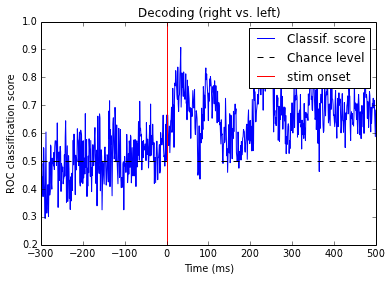Exercise

• Can you improve the performance using full epochs and a common spatial pattern (CSP) used by most BCI systems?

Have a look at the example

http://martinos.org/mne/dev/auto_examples/decoding/plot_decoding_csp_space.html

In []: